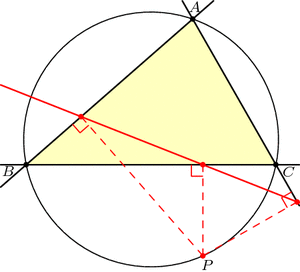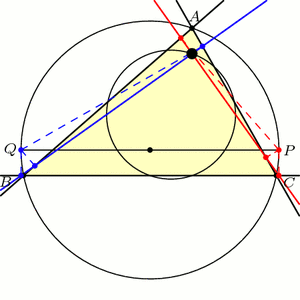# Three-Cornered Deltoids

[This is the third and final post in this series on triangle geometry. See the previous posts on Morley’s theorem and the 9-point circle.]

For our final exploration in this series, let’s again begin with our triangle ABC and a point P on the circumcircle of the triangle, i.e., the circle through the three vertices. If we drop P directly onto the three lines of the triangle at right angles, then by coincidence, these three points lie on a single line, called the Simson line of point P.Just for fun, let’s draw point Q diametrically opposite from P on the circumcircle, and let’s also look at Q‘s Simson line. How do these two Simson lines interact? Somewhat surprisingly, these lines intersect at right angles. The phenomenal part is that this point of intersection lies on the 9-point circle!Furthermore, as P and Q move around the circumcircle, the intersection of their Simson lines moves around the 9-point circle at twice the speed and in the opposite direction:This story gets even more unbelievable when we look at how the Simson lines move in this animation. As it turns out, the Simson lines trace a curve in the shape of a deltoid, which is like an equilateral triangle with curved sides. The deltoid traced here is called Steiner’s deltoid.And finally, here’s an incredible fact that ties everything together: If we draw the equilateral triangle around this deltoid, then the edges are parallel to the edges of the equilateral Morley triangle(s).Holy Morley!

### Notes

1. Note that we may have to extend the lines beyond the triangle. []
2. Specifically, a deltoid is the shape that results when you roll a circle inside a circle three-times larger and trace the path of a single point. See Wikipedia:Deltoid_curve for more information. []

## 3 thoughts on “Three-Cornered Deltoids”

1.Dana Mackenzie says:

I love this! By the way, this is the second example I have seen of a Morley triangle “orienting” a deltoid. I wrote about the first in an obscure Italian journal, “Note di matematica,” vol. 13 no. 2, pp. 237-250 (1993), in an article called “What is the Shape of a Triangle?”
Here’s the setup. Suppose you know that the three vertices of a triangle lie on the unit circle. (Thus the triangle’s circumcenter is at the origin.) What is the locus of possible points for the orthocenter? In this version of the problem, the answer is not too interesting; the locus is a disk of radius 3. However, it turns out that we can get a much nicer answer by specifying a canonical orientation for the triangle. If we require the three vertices to be oriented in such a way that the Morley triangle has an altitude parallel to the x-axis (something that can always be achieved, obviously, by rotating the triangle), then a beautiful result emerges: the orthocenter must lie in a deltoid that is tangent to the unit circle and has vertices at 3, 3 e^(2pi i/3), and 3 e^(4pi i/3). In other words, the deltoid/locus of possible orthocenters is oriented in the same way as the Morley triangle.
One reason that the Morley triangle comes up here is that if the vertices of the triangle are written as complex numbers, z_1, z_2, and z_3, where each z_i has norm equal to 1, then the altitudes of the Morley triangle point in the direction of the three cube roots of (z_1 z_2 z_3)! I was stunned to discover this relationship between the geometry of triangles and the algebra of complex numbers. I proved it in my paper, but unfortunately the only proof I could find was extremely clumsy. It seems to me there ought to be a beautiful proof for this beautiful fact.

1.Zachary Abel says:

This is neat! Thank you for directing me to your paper. It is fascinating how cleanly the orthocenter of a triangle measures seemingly unrelated properties such as “obtuseness”. I wonder if one of these deltoids may be derived from the other?

As for the fact that the Morley triangle altitudes are parallel to the cube roots of z_1 z_2 z_3, here is a sketch of a computation-free geometric proof:

If triangles ABC and AB’C’ are both inscribed in the unit circle and have BC parallel to B’C’, say they are related by a “parallel edge slide” of BC to B’C’.

Lemma: If triangles ABC and AB’C’ are related by parallel edge slide as above, then their morley triangles have parallel corresponding sides.

Proof: If ABC has Morley triangle PQR (as in my Morley Theorem post), then the angle between lines BC and QR can be checked to be $$\gamma-\beta=(\angle C-\angle B)/3$$. Because ABC and AB’C’ share a circumcircle and BC is parallel to B’C’, $$\angle C-\angle B = \angle C’-\angle B’$$. The Lemma follows.

If we interpret the vertices as complex numbers, notice also that $$A\cdot B\cdot C = A\cdot B’\cdot C’$$. Now, any triangle ABC may be manipulated into an equilateral triangle by 2 parallel edge slides: one to make it isosceles and the other to get equilateral. So, by the above observations, we simply have to check the desired theorem for equilateral triangles, which is straightforward.

This method closely follows (and the Lemma is pulled directly from) this paper, which proves existence and some properties of Steiner’s Deltoid: Miguel de Guzmán. “The envelope of the Wallace-Simpson lines of a triangle. A simple proof of the Steiner problem on the deltoid.” RACSAM, 95(1):57–64, 2001.

2.Vishy Anand says:

Very nice contribution… By the way, I love obscure journals if they have the standard of Note di Matematica!